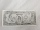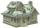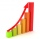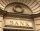# Two accounts

A banker divided $5000 between 2 accounts, one paying 10% annual interest and the second paying 8% annual interest. Express the amount invested in the 10% account in the terms of the amount invested in the 8% account. Result a = (Correct answer is: 5000 - b)### Step-by-step explanation: $a=5000-b$Did you find an error or inaccuracy? Feel free to write us. Thank you!Tips to related online calculators Our percentage calculator will help you quickly calculate various typical tasks with percentages. #### You need to know the following knowledge to solve this word math problem: ## Related math problems and questions: • DepositOh I total of$15,000 deposited into simple interest accounts the annual simple interest rate on one account at 6% the annual simple interest rate on the second account at 7% how much should be invested in each account so that the total interest earned is
• Semiannually compound interestIf you deposit $5000 into an account paying 8.25% annual interest compounded semiannually, how long until there is$9350 in the account?
• Saving 9An amount of $2000 is invested at an interest of 5% per month. If$ 200 is added at the beginning of each successive month but no withdrawals. Give an expression for the value accumulated after n months. After how many months will the amount has accumula
• Compound InterestIf you deposit $6000 in an account paying 6.5% annual interest compounded quarterly, how long until there is$12600 in the account?
• Two accountsTwo accounts in the bank, one per year interest 2%, the second 3%. Total interest income 1900 USD. If interest rates were reversed, the yield would be USD 200 higher. What are the amounts on each account?
• If you 4If you deposit $2500 in an account paying 11% annual interest compounded quarterly, how long until there is the$4500 in the account?
• If you 2If you deposit $4000 into an account paying 9% annual interest compounded monthly, how long until there is$10000 in the account?
• LoanIf you take a bank loan $10000 and we want to repay after the year, we have to pay the total amount$ 10320/ What is the annual interest rate on this loan?
• InterestWhat is the annual interest rate on your account if we put $x and after$n days received $y? • If you 3If you deposit$4500 at 5% annual interest compound quarterly, how much money will be in the account after 10 years?
• Compound interest 43600 dollars is placed in an account with an annual interest rate of 9%. How much will be in the account after 25 years, to the nearest cent?
• SavingsThe depositor regularly wants to invest the same amount of money in the financial institution at the beginning of the year and wants to save 10,000 euros at the end of the tenth year. What amount should he deposit if the annual interest rate for the annua
• Annual pensionCalculate the amount of money generating an annual pension of EUR 1000, payable at the end of the year and for a period of 10 years, shall be inserted into the bank to account with an annual interest rate of 2%
• Investment1000$is invested at 10% compound interest. What factor is the capital multiplied by each year? How much will be there after n=12 years? • Annual interestA loan of 10 000 euro is to be repaid in annual payments over 10 years. Assuming a fixed 10% annual interest rate compounded annually, calculate: (a) the amount of each annual repayment (b) the total interest paid. • Four pupilsFour pupils divided$ 1485 so that the second received 50% less than the first, the third 1/2 less than a fourth, and fourth $154 less than the first. How much money had each of them? • Simple interest 4Find the simple interest if$x USD at $p% for$m days. Assume a \$r-day year.